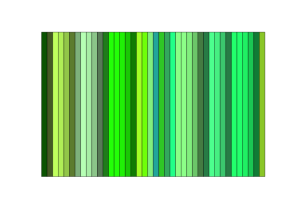# R Color Basics

#### R Color Basics

R graphical devices support a broad range of colors and color functions.  The colors() command with no input arguments returns a list of all available colors in R.  Presently, there are 657 colors.  In practice, declaring colors can be achieved three ways:

• character names (e.g. “red”, “orange”, “yellow”),
• 3-digit RGB values (e.g. 255 0 0265 155 0255 255 0), and
• hexadecimal strings (e.g. “#FF0000”, “#FFA500”, “#FFFF00”).

#### R Color character names

Index extraction from the colors() list is one way to reveal available colors.  For example:

Using color names in plots is straightforward:#### RGB Color Codes in R

The function col2rgb() can be used to extract the RGB (red-green-blue) components of a color.

Each of the RGB color components ranges from 0 to 255.  Given that the three RGB components having 256 possible values, then there are 256*256*256 possible colors, or a total of 16,777,216 colors.

#### Hexadecimal Colors (#rrggbb) in R

Hexadecimal colors represent a more efficient way (for machines) to represent the three RGB colors.  Hexadecimal notation is a number scheme with a base of 16 (or hex) and the primary numbers 0, 1, 2 , 3, 4, 5, 6, 7, 8, 9, A, B, C, D, E, F.

For example, a color with the RGB values red=36, green=104, blue=160 is a greyish-blue color. The decimal numbers 36, 104 and 160 are defined in hexadecimal notation as 24, 68 and A0 respectively. To obtain the hex triplet, we simply concatenate the hash symbol and the three hex bytes together: #2468A0. If a number is less than 16 (in decimal notation) or 10 (in hex notation), it must be represented with a leading zero to keep the number of digits in the triplet equal to six. For example, the decimal triplet 0, 1, 2 would be represented by the hex triplet 000102.

R provide the function as.hexmode() to convert decimal numbers into hex numbers and the rgb() function to convert RGB triplets into hex triplets.

#### R Color Ramps and Hex Triplets

Hex triplets can be used in R in place of color names and are convenient when functions define color selection. For example, it is impossible to use character names to precisely define a color ramp, or the the range of colors that interpolate between a start and end color. Its easy with hex triplets!